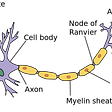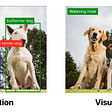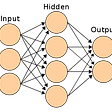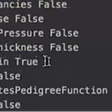# K-Nearest Neighbors Classification

KNN is perhaps one of the simplest ML models out there. It is a non-parametric model, which means it does not make any assumption on underlying data distribution. It is a lazy learning algorithm as it does not learn from the training set immediately instead the learning process is performed at the time of prediction. It can be used for both regression and classification problems.

The underlying logic behind KNN is similarity measure. It measures the similarity between the new uncategorized data and historical categorized data and assigns the new data into categories that is most similar to the pre-defined categories.

KNN follows very basic rule to classify the observations.

In order to measure similarity, distance metrics such as Euclidean distance, Minkowski distance, Jaccard coefficient and Gower’s distance are used, with Euclidean distance being the most popular one.

Considering two observations, O1, and O2 with features as X₁₁, X₁₂…..X₁n and X₂₁, X₂₂….X₂n respectively; we can easily calculate Euclidean distance as,

Let us write some code to see how it works. We’ll be using sklearn’s breast_cancer dataset for our purpose.

`#importing librariesimport pandas as pdimport numpy as npimport matplotlib.pyplot as pltimport seaborn as sns%matplotlib inline#importing data from sklearnfrom sklearn.datasets import load_breast_cancerdata = load_breast_cancer()#converting data into pandas dataframecanc_data = pd.DataFrame(data.data, columns=data.feature_names)#adding target field to the datasetcanc_data['target'] = pd.Series(data.target)#creating X and yX_feature = list(canc_data.columns)X_feature.remove('target')X = canc_data[X_feature]y = canc_data['target']#splitting data for training and testingfrom sklearn.model_selection import train_test_splitX_train, X_test, y_train, y_test = train_test_split(X, y, test_size = 0.3, random_state = 100)`

Now that we have the dataset ready, we can implement KNN algorithm on it.

`#importing KNN from sklearnfrom sklearn.neighbors import KNeighborsClassifierknn_clf = KNeighborsClassifier(n_neighbors=5, metric='minkowski').fit(X_train, y_train)#predicting for our test datay_pred = knn_clf.predict(X_test)#generating classification reportfrom sklearn.metrics import classification_reportprint(classification_report(y_test, y_pred))#importing libraries to check model performancefrom sklearn.metrics import confusion_matrixfrom sklearn.metrics import accuracy_scorefrom sklearn.metrics import log_lossfrom sklearn import metricsprint("Accuracy score on test: " , round((knn_clf.score(X_test, y_test)),3))print("Accuracy score on train: ", round((knn_clf.score(X_train, y_train)),3))#printing log loss for the modelprint('log_loss : ', log_loss(y_test, y_pred))#let find ROC and AUC score#before we calculate roc_auc_score(), we need to find out the predicted probabilities for test data.pred_prob = pd.DataFrame(knn_clf.predict_proba(X_test))#we'll also add the actual labeltest_result = pd.DataFrame( { 'actual' : y_test})test_result = test_result.reset_index()test_result['prob_0'] = pred_prob.iloc[:,0:1]test_result['prob_1'] = pred_prob.iloc[:,1:2]#to calculate ROC AUC score we will pass actual class labels and predicted probabilityauc_score = round(metrics.roc_auc_score(test_result.actual, test_result.prob_1),3)print("AUC Score : ",auc_score)#generating confusion matrixcf_matrix = confusion_matrix(y_test, y_pred)sns.heatmap(cf_matrix, annot=True, cmap='Blues')plt.ylabel("True Label")plt.xlabel("Predicted Label")plt.show()`

We can also perform hyperparameter tuning to search for optimal parameters. Using GridSearchCV from sklearn we can perform search from a list of hyperparameter values for optimal model efficiency.

GrisSearchCV will take the following parameters :

1. estimator : The ml model
2. param_grid : A dictionary with parameter names as key and list of parameter values as values.
3. scoring : accuracy measure. ‘r2’ for regression, ‘recall’, ‘precision’ ,etc. for classification.
4. cv : number of folds in k-fold (Each set of values will be evaluated by K-fold cross validation, thus the final score will be the average of score from all folds of K-fold cross validation)
`#hyperparameter tuning using grid searchfrom sklearn.model_selection import GridSearchCV#we'll create a dictionary with possible hyperparameter valuesparam_val = [{'n_neighbors' : range(3,10), 'metric' : ['euclidean', 'minkowski', 'canberra']}]#grid search configurationclfr = GridSearchCV(KNeighborsClassifier(), param_val, cv = 10, scoring = 'roc_auc')#fitting into our dataclfr.fit(X_train, y_train)`

Once we have run the GridSearchCV , we can the see for the best parameters as follows:

`#we'll see for best score and parametersprint(clfr.best_score_)print(clfr.best_params_)`

We can use accuracy plot as well to see how the accuracy of model varies with different values of k.

`# creating empty list variableacc = []# running KNN algorithm for 3 to 50 nearest neighbours(odd numbers) and storing the accuracy valuesfor i in range(3,50,2):  neigh = KNeighborsClassifier(n_neighbors=i)  neigh.fit(X_train, y_train)  train_acc = np.mean(neigh.predict(X_train) == y_train)  test_acc = np.mean(neigh.predict(X_test) == y_test)  acc.append([train_acc, test_acc])import matplotlib.pyplot as plt # library to do visualizations# train accuracy plotplt.plot(np.arange(3,50,2),[i for i in acc],"ro-")plt.show()`

--

--

--

## More from Ankit Gupta

Love podcasts or audiobooks? Learn on the go with our new app.

## Recommended from Medium## Analysis of Tweets about the Joker (2019 film) in Python## An Introduction to Neural Networks — The Perceptron## NLP: Building Text Summarizer — Part 2## Tracking the Millennium Falcon with TensorFlow## An Intuitive Guide To Understanding The Learning Process Of A Neural Network## Automating Plant Recognition## Binary Classification with Logistic Regression## Deep Dive EDA on IRIS Dataset## Used Car Price prediction & analysis## Diabetes Prediction Using Machine Learning Algorithms